# Regular Expressions

## Overview

Finite automata provide one way of specifying regular languages.

Another way of specifying a regular language is to write a regular expression.

An example regular expression appears below:

$(0 \cup 1) 0^\ast$

This regular expression specifies the regular language $\{w \;|\; w \text{ consists of a zero or one followed by any number of zeroes}\}$

$L(R)$ refers to the regular language of the regular expression R.

This refers to classical regular expressions. Programming languages, editors and textual tools support extended regular expressions, which include more features than we will discuss here.

## Formal Definition

$R$ is a regular expression if $R$ is one of:

1. $a \text{ for some } a \in \Sigma$
2. $\epsilon$
3. $\emptyset$
4. $(R_{1} \cup R_{2}) \text{ where } R_{1} \text{ and } R_{2} \text{ are regular expressions}$
5. $(R_{1} \circ R_{2}) \text{ where } R_{1} \text{ and } R_{2} \text{ are regular expressions}$
6. $(R^\ast) \text{ where } R \text{ is a regular expression}$.

This is an inductive definition. It defines a regular expression in terms of smaller expressions until it's broken down to individual characters.

Parenthesis can be omitted, and the order of precedence is: star, concatenation, union.

We usually drop the $\circ$ operator. For example $a \circ b$ is usually just written $ab$.

For convenience, we define $R^+ = RR^\ast$.

We also allow $\Sigma$ to appear in a regular expression which means any single element of the alphabet.

What language do each of the following regular expressions produce?

1. $0^\ast1^\ast$
2. $(01)^\ast$
3. $\Sigma^+$
4. $(00 \cup 11)^\ast$
5. $0^+1^+0^+$

## Equivalence with Finite Automata

Regular expressions produce languages in the same class that our automata can recognize, the regular languages.

To show the two are equivalent, we need to show that:

1. Any regular expression can be converted to an equivalent NFA or DFA.
2. Any NFA or DFA can be converted into an equivalent regular expression.

## Regular Expression $\rightarrow$ NFA

The definition of a regular expression includes 6 cases. We will consider each one in turn.

1. $R = a \text{ for some } a \in \Sigma$. $L(R) = \{a\}$, and the following NFA recognizes $L(R)$: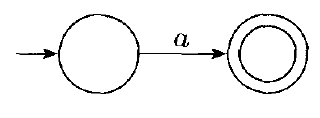2. $R = \epsilon$. $L(R) = \{\epsilon\}$, and the following NFA recognizes $L(R)$: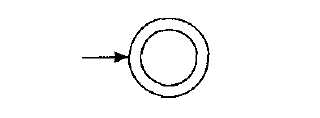3. $R = \emptyset$. $L(R) = \emptyset$, and the following NFA recognizes $L(R)$: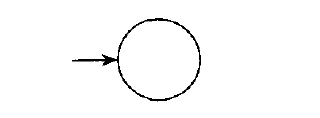4. $R = R_{1} \cup R_{2}$. For this case, we use the same construction which shows how to build an NFA recognizing union: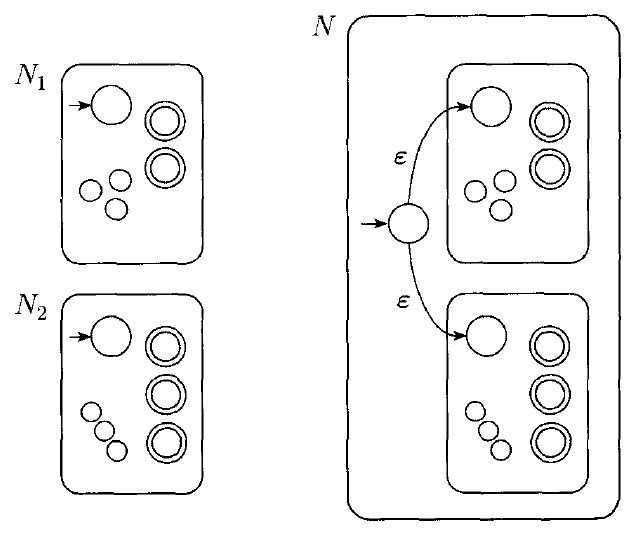5. $R = R_{1} \circ R_{2}$. For this case, we use the same construction which shows how to build an NFA recognizing concatenation: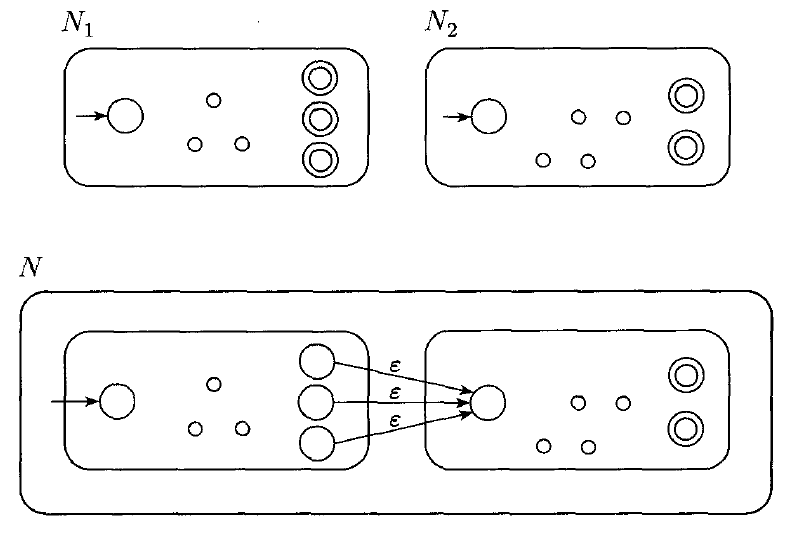6. $R = R_{1}^\ast$. For this case, we use the same construction which shows how to build and NFA recognizing star: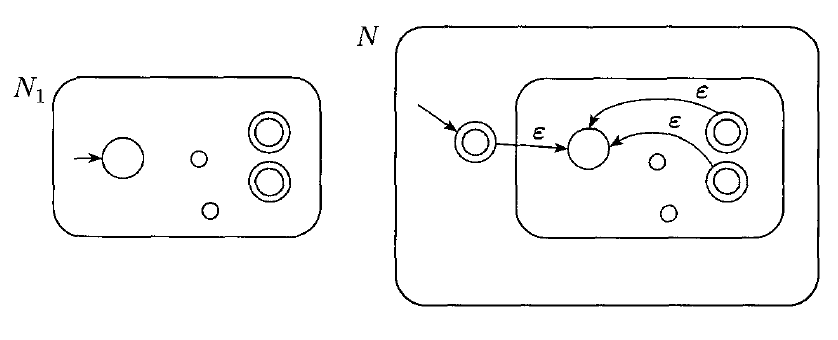For each regular expression, we can use the definition of a regular expression to guide our construction of an equivalent NFA.

This shows that any regular expression can be converted into an NFA recognizing the same language. We still must show the reverse, that any NFA can be converted into an equivalent regular expression.

## Example Conversion

Can we use this construction technique to convert the regular expression $(ab \cup a)^\ast$ into an NFA?